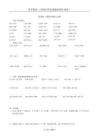95%的用户最终下载了:

约有235331篇,以下是第1-10篇排序：相关性|最多阅读|最新上传只显示优质文档只显示可投资文档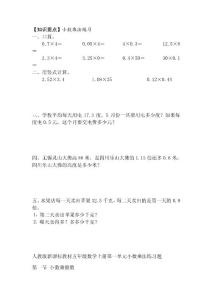55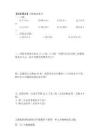【知识要点】小数乘法练习一、口算。 0.74＝ 0.054＝ 40.3＝ 12.58 2.34＝3.250＝ 0.450＝ 300.1 二、用竖式计算。2.523.4 1.0825 0.120.44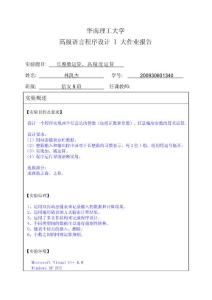68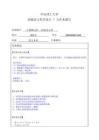42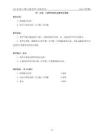2002学年第一学期小学数学第十一册备课笔记 爱山小学陈国权 118 四、分数、小数四则混合运算和应用题 教学内容： 1、四则混合运算 2、两步计算的分数（百分数）应用题 教学目标： 1、使学生能正确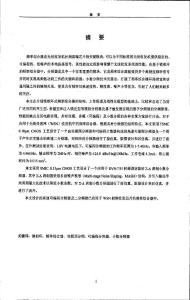94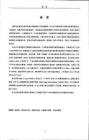PLL频率综合器中整数和小数分频器设计与实现.pdf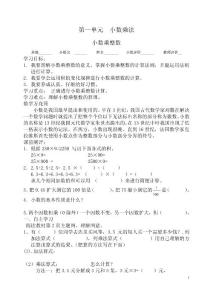98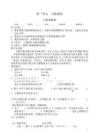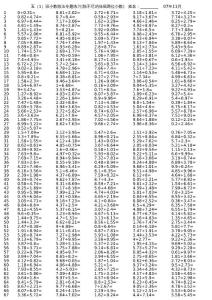39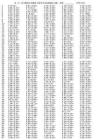00.31=8.612.02= 2.726.71= 3.181.61= 3.724.25= 0.824.74=0.78.4= 0.592.93= 9.173.67= 7.743.27= 6.978.4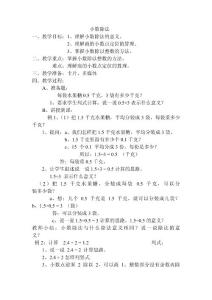61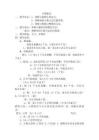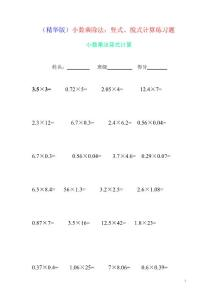134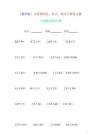(精华版)小数乘除法:竖式、脱式计算练习题.doc
（精华版）小数乘除法：竖式、脱式计算练习题: 小数乘法竖式计算3.5×3= 0.72×5= 2.05×4= 12.4×7= 2.3×12= 6.7×0.3= 2.4×6.2= 0.56×0.04= 6.7×0.3= 0.56×0.04= 3.7×4.6= 0.29×0.07= 6.5×8.4= 56×1.3= 3.2×2.5= 2.6×1.08= 0.87×7= 3.5×16= 12.5×42= 1.8×23= 0.37×0.4= 1.06×25= 7×8.06= 0.6×0.39=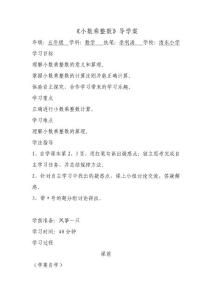188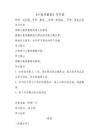《小数乘整数》导学案年级：五年级 学科：数学 执笔：李利涛 学校：清东小学 学习目标 理解小数乘整数的意义和算理。 掌握小数乘整数的计算法则并能正确计算。 体验自主探究、合作学习带来的学习乐趣。 学习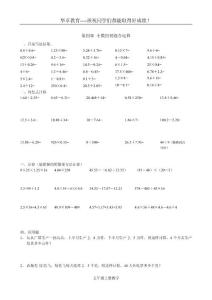40